Mathematical and Physical Journal
for High Schools
Issued by the MATFUND Foundation
 Already signed up? New to KöMaL?

# Exercises and problems in PhysicsApril 2003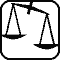## Experimental problem

M. 242. Measure the freezing point of the aqueous solution of glycerol as the function of the degree of dilution (mass percentage of glycerol).

(6 points)## Theoretical problems

It is allowed to send solutions for any number of problems, but final scores of students of grades 9-12 are computed from the 5 best score in each month. Final scores of students of grades 1-8 are computed from the 3 best scores in each month.

P. 3611. Consider a pizza as a thin, homogeneous disk of radius r and mass m. We have already cut out and eaten a 20o slice of it. By what percentage of the radius has the center of the mass of the pizza shifted?

(3 points)

P. 3612. A bucket hung at the end of a rope is moving as a pendulum. Does the swinging period increase or decrease if the water in the bucket drips away through a hole at the bottom of the bucket?

(5 points)

P. 3613. We have prepared a closed ring of iron wire and soldered two other wires on the end-points of one of its quarter circles as shown in the figure. How much current flows in the wires leading to the ring if the resistance of a quarter circle is 0.2 $\displaystyle \Omega$, and between the soldered points a voltage of 0.6 V can be measured?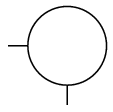(3 points)

P. 3614. Estimate in what thickness could the dry lands of the earth be covered by the wheat grains that the alleged inventor of chess asked from the king as a reward. (He asked one grain on the first square and asked to double the number of grains on every subsequent square.)

(4 points)

P. 3615. On a horizontal surface two bodies of the same mass start at the same time towards each other from a 4 m distance and collide non-elastically. The initial velocity of the right side body, moving with constant velocity, is the double of the initial velocity of the left side body, which moves with constant acceleration of 0.5 m/s2. a) What are the initial velocities? b) What percentage of the mechanical energy is lost in the non-elastic collision?

(4 points)

P. 3616. The air pressure in a cylinder closed by a piston increases proportionally to the cylinder volume. In the process the air absorbs heat of 90 kJ. How much work is done by the expanding gas, and by what amount does its energy increase?

(4 points)

P. 3617. A capacitor charged up to a voltage of 100 V is discharged through a resistance. The discharging current decreases by 60% every 2 seconds from the initial 100 $\displaystyle \mu$A. a) What is the discharging resistance? b) What is the capacity of the capacitor? c) After how much time does the current decrease to 0.41 $\displaystyle \mu$A?

(5 points)

P. 3618. Two infinite plates of thickness d and of volume charge densities of +$\displaystyle \varrho$ and -$\displaystyle \varrho$ are placed next to each other. Calculate and plot the electric field strength and potential as a function of the distance from the plates.

(5 points)

P. 3619. An electron beam enters a homogeneous magnetic field where the Lorentz force makes it circle with a radius of R. The width'' of the magnetic field is $\displaystyle \ell$=R/5 and in a L=5R distance from it there is a fluorescent screen where the electrons impact on. What is the deflection of the electron beam on the screen? What would happen if the accelerating voltage was decreased to its a) fourth? b) twenty-fifth?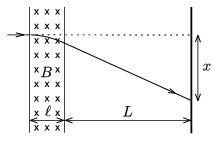(4 points)

P. 3620. Two rubber threads of spring constant of 10 N/m each is connected to the two sides of a body of a mass of 1 kg standing on a horizontal frictionless surface. The rubber threads are stretched by a length of $\displaystyle \Delta$and their ends are fixed. Then the body is displaced by a distance of 2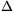towards one of the fixing points and it is released. Determine the period of the resulting oscillating motion.

(5 points)

P. 3621. There is gas in a cylindrical vessel closed up with a piston. The wall of the cylinder is made of insulator material, but its base and the piston is made of metal and behaves like a plate capacitor. Initially the distance of the plates is d0 and the pressure of the enclosed gas is the same as the air pressure outside. How does the distance of the plates change if the capacitor'' is charged up to a voltage U? Assume that the temperature of the gas is constant.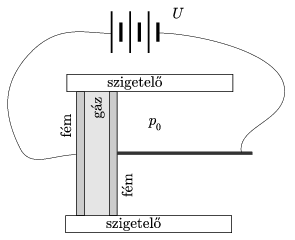(5 points)